# How to Add Percentage Symbol for Multiple Numbers in Cells in Excel?

When you have to add a Percentage symbol to an existing set of data in Excel, you have two ways to do so. The first one is to select the data and click the Percentage symbol under the Home menu and the other method is to select the cells and format cells for percentage. These two methods will not only add the "%" symbol but also multiply the numbers with 100. This tutorial will help you understand how you can add "%" symbol without changing the value of the numbers.

## How to Add Percentage Symbol for Multiple Numbers in Excel

Let us take a simple example to understand how you can add "%" symbol for multiple numbers in Excel.

### Step 1

Let us assume we have the following Excel sheet with the given data −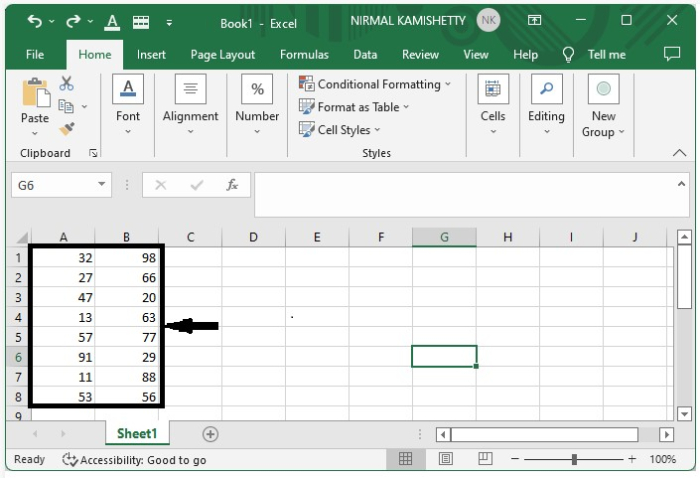Now, click an empty cell where you want to get the first result and enter the formula as "=Address of data/100" as we calculate percentage out of 100.

In our case, we will be using the formula as "A1/100" in the cell "D2" and then press the "OK" button to get the first result, as shown below −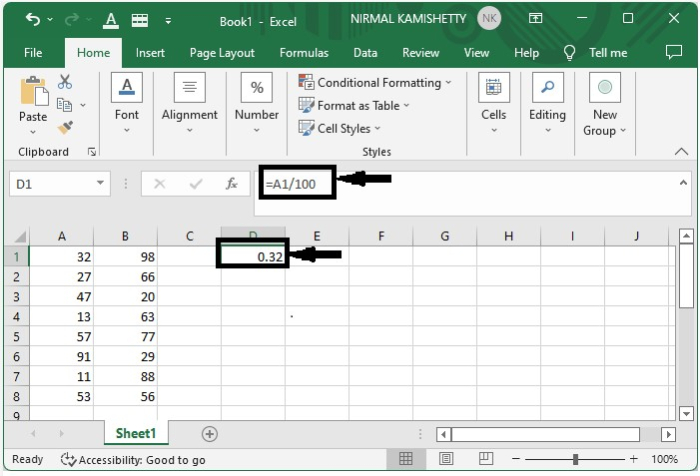### Step 2

Now we need to drag from the cell "D2" till all the results are displayed. The screen will now appear like the one given below −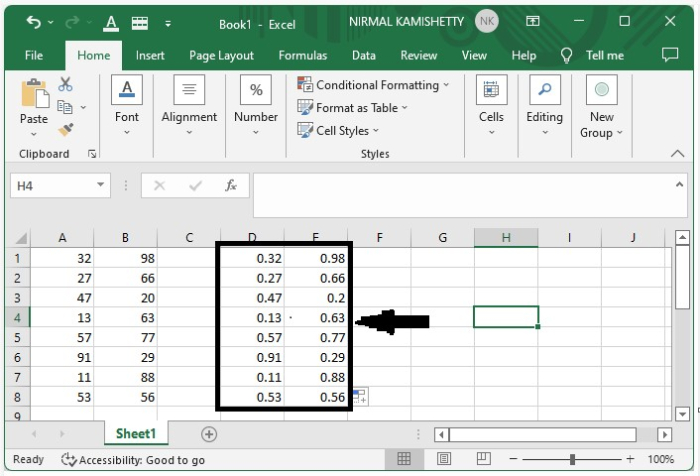### Step 3

To add the "%" symbol, we need to select the data and click "Number" under "Home" menu and then click the Percentage symbol. Select data → home → number → % symbol.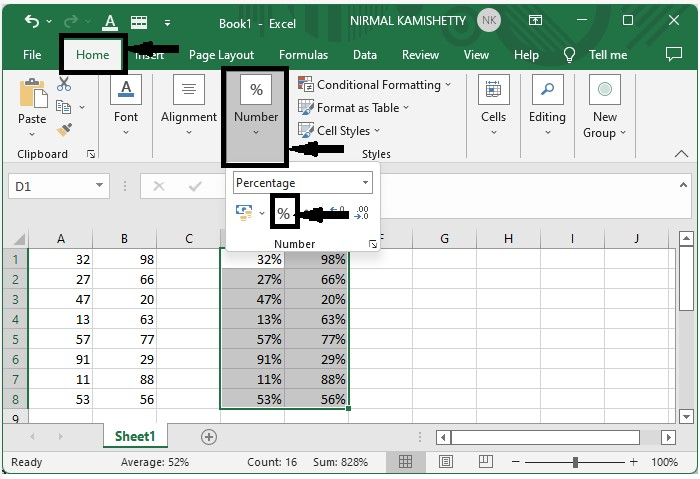The values are added with the "%" symbols successfully, as shown in the screenshot below −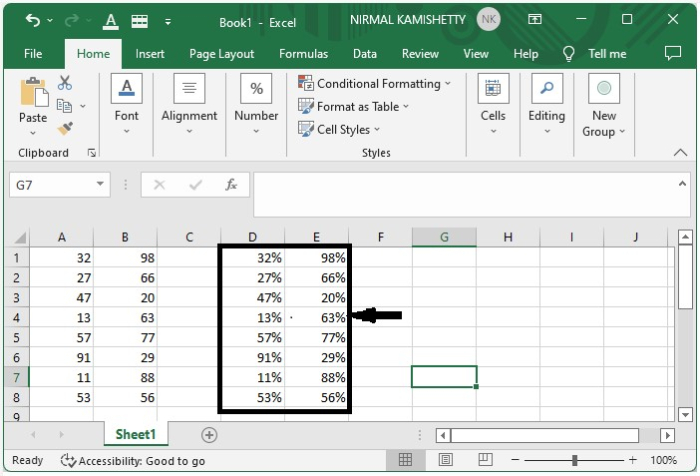We can also use the Format option for the newly generated data to add the "%" symbol. This is how we can add the Percentage symbol in multiple cells in Excel.